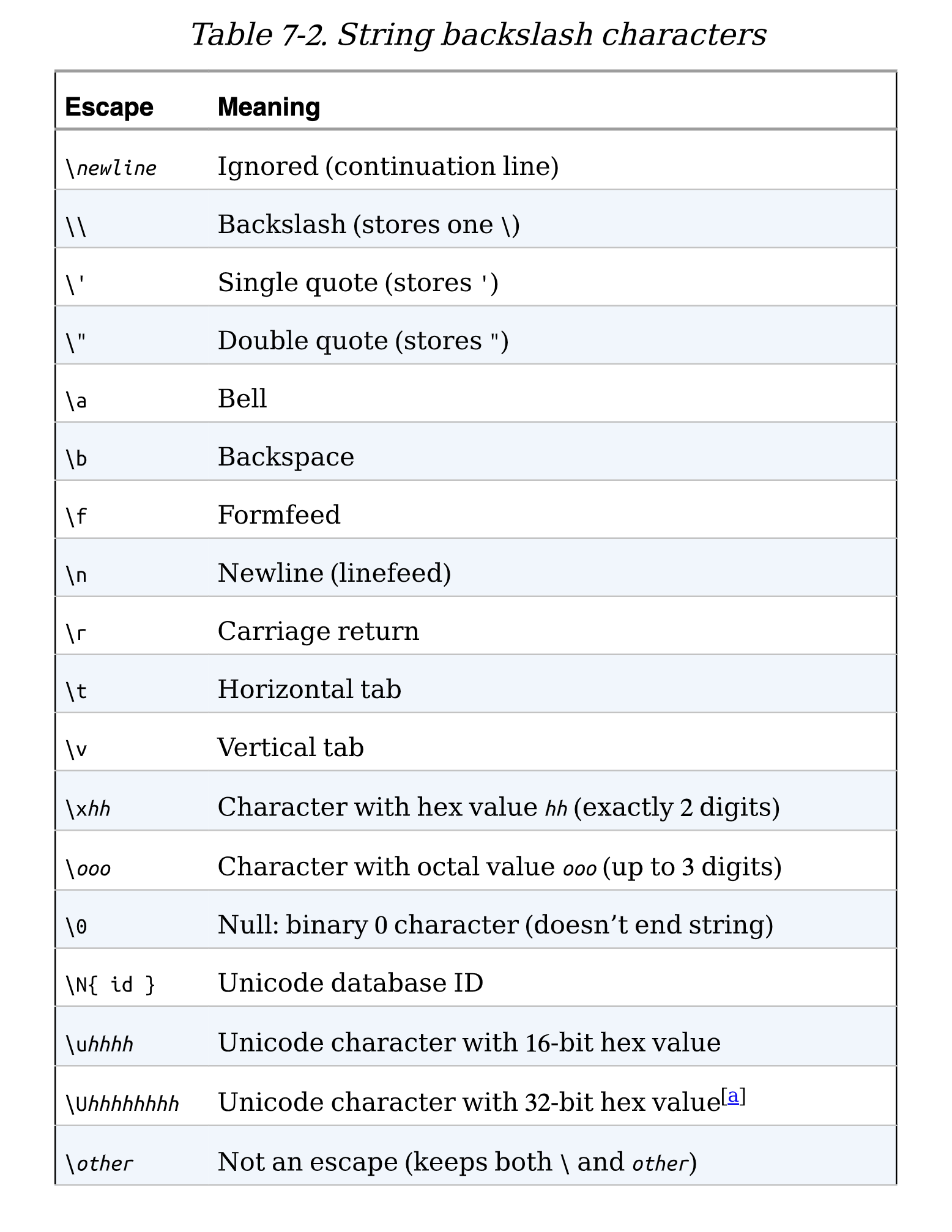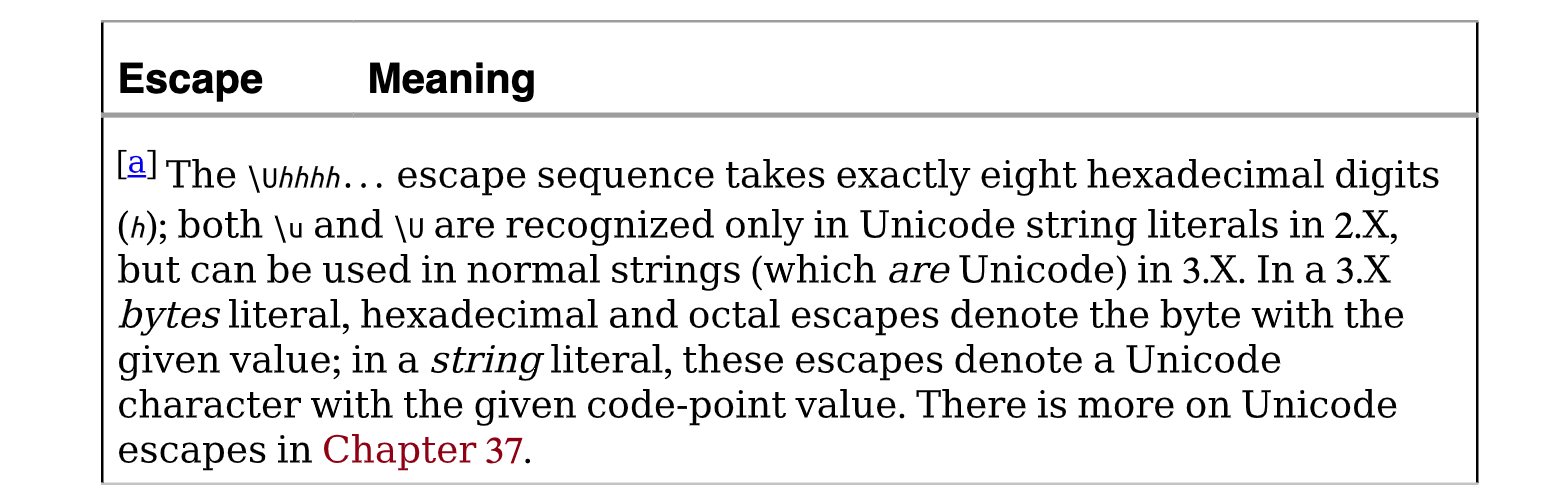# 字符串字面量——转义序列代表特殊字符

``>>> s = 'a\nb\tc'``

``````>>> s
'a\nb\tc'
>>> print(s)
a
b c``````

``````>>> len(s)
5````````````>>> s = 'a\0b\0c'
>>> s
'a\x00b\x00c'
>>> len(s)
5``````

``````>>> s = '\001\002\x03'
>>> s
'\x01\x02\x03'
>>> len(s)
3``````

``````>>> S = "s\tp\na\x00m"
>>> S
's\tp\na\x00m'
>>> len(S)
7
>>> print(S)
s p
a m``````

``````>>> x = "C:\py\code" # 原封不动保留 \  (并将其显示为 \\)
>>> x
'C:\\py\\code'
>>> len(x)
10``````